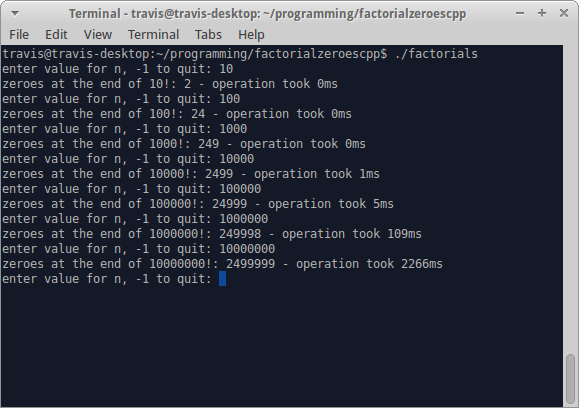# Factorial Zeroes 2

In my last post I talked about finding the number of zeroes at the end of$n!$, and I said that there was room for improvement. I thought about it a little bit and found a couple things to speed it up.

The first has to do with the relationship between the quantity of fives and the quantity of twos. The lower quantity in the prime factorization of$n!$ is how many zeroes it will have at the end. If I would have thought a little more about it though I would have seen that counting the twos is pointless in this situation.

Even the prime factorization of$10 = 5 \cdot 2$ has the information in there: there will always be more twos than fives. Counting from 1 to 10:

• Multiples of 2: 2, 4, 6, 8, 10
• Multiples of 5: 5, 10

This means that all we really need to keep track of is the quantity of fives in the prime factorization of$n!$. Which leads to the second optimization: we only need to get the prime factorization of multiples of five.

Also since we’re only keeping track of the quantity of one number instead of two, we can just keep track of it with a single int instead of a list of ints containing all the prime factorizations in$n!$. That’ll save a whole lot of memory.

I’ve decided to switch it up and write this one in C++, here’s a screenshot of the results:109ms for the Linux/C++ version vs 855ms for the Windows/C# version when calculating the zeroes at the end of$1000000!$. That’s a pretty decent improvement, although surely the language and environment played a factor, but at least it was on the same computer.

I’ve posted the code in a Gist. I won’t post all the code here but I’ll post some of the parts worth noting.

int CountZeroes(int n)
{
if (n < 5)
return 0;

int fives = 1;
int i = 10;
while (i <= n)
{
//i is already a multiple of 5, skip that step
fives += 1 + CountFivesInFactorization(i/5);
i += 5;
}
return fives;
}


This is the method that iterates from 1 to$n$. Notice on line 11 it passes in i/5 as the param to CountFivesInFactorization(). Since we already know i is a multiple of 5 it would be a waste of cycles finding that fact out, so it gets skipped.

int CountFivesInFactorization(int n)
{
if (IsPrime(n))
return n == 5 ? 1 : 0;

for (int i = 2; i*i <= n; i++)
{
if (n % i == 0)
{
int result = n / i;
int fives = 0;

if (IsPrime(result))
{
if (result == 5)
fives++;
}
else
fives += CountFivesInFactorization(result);

if (IsPrime(i))
{
if (i == 5)
fives++;
}
else
fives += CountFivesInFactorization(i);

return fives;
}
}
return 0;
}


Despite being faster at what it was made for that’s not to say the C# version is pointless. This version doesn’t actually keep the prime factorization of$n!$, and that list of numbers has many more uses than just what I’ve used them for. Maybe figuring out a new use for that list can be a future project.